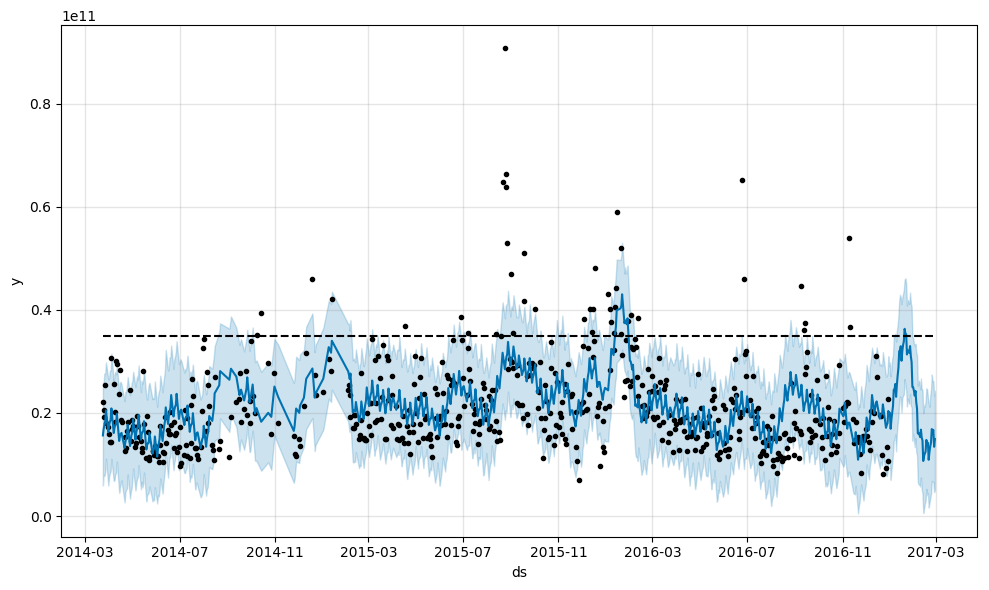# Prophet - Time series prediction

### Brief history

Time series prediction is one of the most common statistical problems over the last 30-50 years. AutoRegressive (AR) models and Moving Average (MA) models were some of the earliest models to be used for time series prediction.

AutoRegressive Moving Average (ARMA) and AutoRegressive Integrated Moving Average (ARIMA) models became more popular over the years.

statsmodels is a great library for the above time series models.

### Prophet

Prophet is a new tool from Facebook that uses stan models for prediction.

### Show me the code!

``````from fbprophet import Prophet
import pandas as pd

# Prophet expects the days/time to be in the 'ds' column and the values in the 'y' column.
df = df.rename(columns={'Unnamed: 0': 'ds', 'SPY': 'y'})

# Fit the Prophet model
df['cap'] = 3.5 * 1e10
m = Prophet(growth='logistic')
m = Prophet()
m.fit(df)

# Predict the notional volume for the next 60 days
future = m.make_future_dataframe(periods=60)
future['cap'] = 3.5 * 1e10
forecast = m.predict(future)
forecast[['ds', 'yhat', 'yhat_lower', 'yhat_upper']].tail()

# Plot
m.plot(forecast)
``````### Notebook

Here is a link to the notebook I added for the same example.